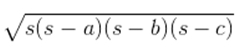Guru

# Find the area of a triangle two sides of which are 18 cm and 10 cm and the perimeter is 42cm. Q.4

• 0

How i solve the question of class 9th ncert math of Heron’s Formula chapter of exercise 12.1 of question no 4. I think it is very important question of class 9th give me the tricky way for solving this question Find the area of a triangle two sides of which are 18 cm and 10 cm and the perimeter is 42cm.

Share

1. Assume the third side of the triangle to be “x”.

Now, the three sides of the triangle are 18 cm, 10 cm, and “x” cm

It is given that the perimeter of the triangle = 42cm

So, x = 42-(18+10) cm = 14 cm

∴ The semi perimeter of triangle = 42/2 = 21 cm

Using Heron’s formula,

Area of the triangle,

== √[21(21-18)(21-10)(21-14)] cm2

= √[21×3×11×7] m2

= 21√11 cm2

• 0